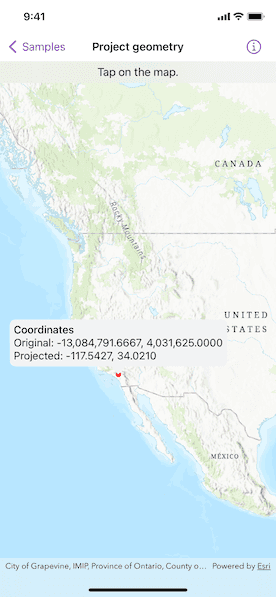# Project geometry

Project a point to another spatial reference.## Use case

Being able to project between spatial references is fundamental in GIS. An example of when you would need to re-project data is if you had data in two different spatial references, but wanted to perform an intersect analysis with the `static GeometryEngine.intersection(_:_:)` method. This method takes two geometries as parameters, and both geometries must be in the same spatial reference. If they are not, you could first use `static GeometryEngine.project(_:into:)` to convert the geometries so they match.

## How to use the sample

Tap anywhere on the map. A callout will display the tapped location's coordinate in the original basemap's spatial reference `webMercator` and in the projected spatial reference `wgs84`.

## How it works

1. Call the method, `static GeometryEngine.project(_:into:)`, passing in the original geometry and a spatial reference system to be projected to.

## Relevant API

• GeometryEngine
• Point
• SpatialReference
• static GeometryEngine.project(_:into:)

In cases where the output spatial reference uses a different geographic coordinate system than that of the input spatial reference, see the `static GeometryEngine.project(_:into:datumTransformation:)` method that additionally takes in a `DatumTransformation` parameter.

## Tags

coordinate system, coordinates, latitude, longitude, projected, projection, spatial reference, Web Mercator, WGS 84

## Sample Code

ProjectGeometryView.swift
Use dark colors for code blocksCopy
``````// Copyright 2022 Esri
//
// you may not use this file except in compliance with the License.
// You may obtain a copy of the License at
//
//
// Unless required by applicable law or agreed to in writing, software
// WITHOUT WARRANTIES OR CONDITIONS OF ANY KIND, either express or implied.
// See the License for the specific language governing permissions and

import ArcGIS
import SwiftUI

struct ProjectGeometryView: View {
/// A location callout placement.
@State private var calloutPlacement: CalloutPlacement?

/// The point where the map was tapped in its original spatial reference (Web Mercator).
@State private var originalPoint: Point!

/// The projected location after normalization.
@State private var projectedPoint: Point!

/// The view model for the sample.
@StateObject private var model = Model()

var body: some View {
MapView(map: model.map, graphicsOverlays: [model.graphicsOverlay])
.onSingleTapGesture { _, mapPoint in
if calloutPlacement == nil {
// Sets the original point to where the map was tapped.
originalPoint = GeometryEngine.normalizeCentralMeridian(of: mapPoint) as? Point

// Projects the original point from Web Mercator to WGS 84.
projectedPoint = GeometryEngine.project(originalPoint!, into: .wgs84)!

// Updates the geometry of the point graphic.
model.pointGraphic.geometry = projectedPoint

// Updates the location callout placement.
calloutPlacement = CalloutPlacement.location(projectedPoint)
} else {
// Hides the callout and point graphic.
calloutPlacement = nil
model.pointGraphic.geometry = nil
}
}
.callout(placement: \$calloutPlacement.animation(.default.speed(2))) { _ in
Group {
Text("Coordinates")
.fontWeight(.medium)
Text("Original: \(originalPoint.xyCoordinates)")
Text("Projected: \(projectedPoint.xyCoordinates)")
}
.font(.callout)
}
}
.overlay(alignment: .top) {
Text("Tap on the map.")
.frame(maxWidth: .infinity)
.background(.thinMaterial, ignoresSafeAreaEdges: .horizontal)
}
}
}

private extension ProjectGeometryView {
/// The model used to store the geo model and other expensive objects
/// used in this view.
class Model: ObservableObject {
/// A map with a topographic basemap style and an initial viewpoint.
let map: Map = {
let map = Map(basemapStyle: .arcGISTopographic)
map.initialViewpoint = Viewpoint(
center: Point(x: -1.2e7, y: 5e6, spatialReference: .webMercator),
scale: 4e7
)
return map
}()

/// A graphics overlay containing a graphic with a circular, red marker symbol.
let graphicsOverlay = GraphicsOverlay(graphics: [
Graphic(symbol: SimpleMarkerSymbol(color: .red, size: 8))
])

/// The graphic with a circular, red marker symbol.
var pointGraphic: Graphic { graphicsOverlay.graphics.first! }
}
}

private extension FormatStyle where Self == FloatingPointFormatStyle<Double> {
/// Formats the double with four decimals places of precision.
static var decimal: Self {
Self.number.precision(.fractionLength(4))
}
}

private extension Point {
/// The point's decimal-formatted x and y coordinates.
var xyCoordinates: Text {
Text("\(self.x, format: .decimal), \(self.y, format: .decimal)")
}
}``````